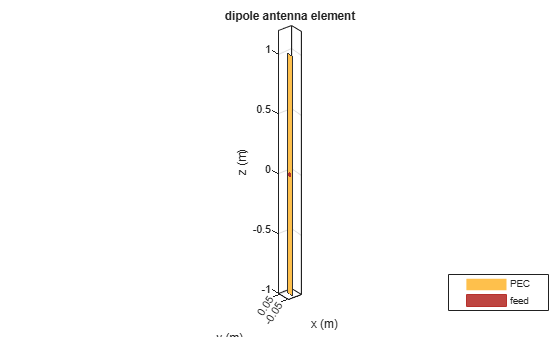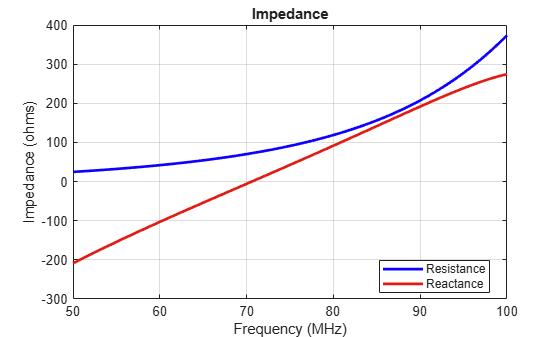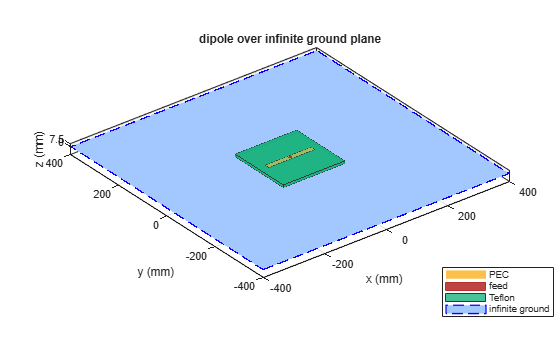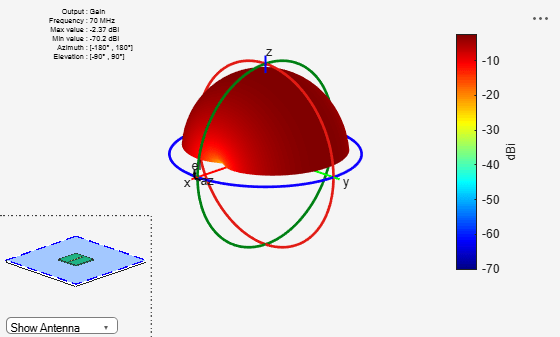Documentation

dipole

Create strip dipole antenna

Description

The dipole object is a strip dipole antenna on the Y-Z plane.

The width of the dipole is related to the diameter of an equivalent cylindrical dipole by the equation

$w=2d=4r$

where:

• d is the diameter of equivalent cylindrical dipole.

• r is the radius of equivalent cylindrical dipole.

For a given cylinder radius, use the cylinder2strip utility function to calculate the equivalent width. The default strip dipole is center-fed. The feed point coincides with the origin. The origin is located on the Y-Z plane.Creation

Description

d = dipole creates a half-wavelength strip dipole antenna on the Y-Z plane.

example

d = dipole(Name,Value) creates a dipole antenna, with additional properties specified by one or more name-value pair arguments. Name is the property name and Value is the corresponding value. You can specify several name-value pair arguments in any order as Name1, Value1, ..., NameN, ValueN. Properties you do not specify retains their default values.

Properties

expand all

Dipole length, specified as a scalar in meters. By default, the length is chosen for an operating frequency of 75 MHz.

Example: 'Length',3

Data Types: double

Dipole width, specified as a scalar in meters.

Note

Dipole width should be less than 'Length'/5 and greater than 'Length'/1001. 

Example: 'Width',0.05

Data Types: double

Signed distance from center of dipole, specified as a scalar in meters. The feed location is on Y-Z plane.

Example: 'FeedOffset',3

Data Types: double

Lumped elements added to the antenna feed, specified as a lumped element object handle. For more information, see lumpedElement.

Example: 'Load',lumpedelement. lumpedelement is the object handle for the load created using lumpedElement.

Example: d.Load = lumpedElement('Impedance',75)

Tilt angle of the antenna, specified as a scalar or vector with each element unit in degrees. For more information, see Rotate Antennas and Arrays.

Example: 'Tilt',90

Example: 'Tilt',[90 90],'TiltAxis',[0 1 0;0 1 1] tilts the antenna at 90 degree about two axes, defined by vectors.

Data Types: double

Tilt axis of the antenna, specified as:

• Three-element vectors of Cartesian coordinates in meters. In this case, each vector starts at the origin and lies along the specified points on the X-, Y-, and Z-axes.

• Two points in space, each specified as three-element vectors of Cartesian coordinates. In this case, the antenna rotates around the line joining the two points in space.

• A string input describing simple rotations around one of the principal axes, 'X', 'Y', or 'Z'.

For more information, see Rotate Antennas and Arrays.

Example: 'TiltAxis',[0 1 0]

Example: 'TiltAxis',[0 0 0;0 1 0]

Example: ant.TiltAxis = 'Z'

Object Functions

 show Display antenna or array structure; Display shape as filled patch info Display information about antenna or array axialRatio Axial ratio of antenna beamwidth Beamwidth of antenna charge Charge distribution on metal or dielectric antenna or array surface current Current distribution on metal or dielectric antenna or array surface design Design prototype antenna or arrays for resonance at specified frequency EHfields Electric and magnetic fields of antennas; Embedded electric and magnetic fields of antenna element in arrays impedance Input impedance of antenna; scan impedance of array mesh Mesh properties of metal or dielectric antenna or array structure meshconfig Change mesh mode of antenna structure pattern Radiation pattern and phase of antenna or array; Embedded pattern of antenna element in array patternAzimuth Azimuth pattern of antenna or array patternElevation Elevation pattern of antenna or array returnLoss Return loss of antenna; scan return loss of array sparameters S-parameter object vswr Voltage standing wave ratio of antenna

Examples

collapse all

Create and view a dipole with 2m length and 0.5m width.

d = dipole('Width',0.05)
d =
dipole with properties:

Length: 2
Width: 0.0500
FeedOffset: 0
Tilt: 0
TiltAxis: [1 0 0]

show(d)Calculate the impedance of a dipole over a frequency range of 50MHz - 100MHz.

d = dipole('Width',0.05);
impedance(d,linspace(50e6,100e6,51))Design a dipole antenna backed by a dielectric substrate and an infinite reflector.

Create a dipole antenna of length, 0.15 m, and width, 0.015 m.

d = dipole('Length',0.15,'Width',0.015, 'Tilt',90,'TiltAxis',[0 1 0]);

Create a reflector using the dipole antenna as an exciter and the dielectric, teflon as the substrate.

t = dielectric('Teflon')
t =
dielectric with properties:

Name: 'Teflon'
EpsilonR: 2.1000
LossTangent: 2.0000e-04
Thickness: 0.0060

For more materials see catalog
rf = reflector('Exciter',d,'Spacing',7.5e-3,'Substrate',t);

Set the groundplane length of the reflector to inf. View the structure.

rf.GroundPlaneLength = inf;
show(rf)Calculate the radiation pattern of the antenna at 70 MHz.

pattern(rf,70e6)Balanis, C.A. Antenna Theory: Analysis and Design. 3rd Ed. New York: Wiley, 2005.

 Volakis, John. Antenna Engineering Handbook, 4th Ed. New York: Mcgraw-Hill, 2007.